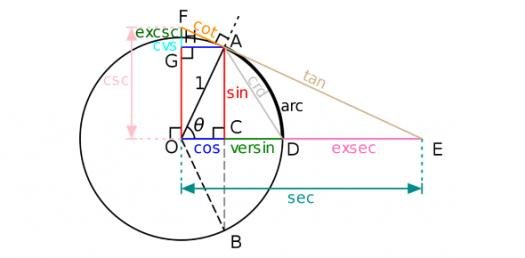# IT's Time To Solve Trigonometry Questions

7 Questions | Total Attempts: 193SettingsYou may need paper, pencil and a calculator.

• 1.
Which rule can you apply when you are given 2 sides and an included angle of a triangle?
• 2.
Will this case give rise to an ambiguity when using Sine rule: given C = 750, a = 9 and c = 11?
• 3.
Will this case give rise to an ambiguity when using Sine rule: given A = 680, a = 12 and b = 9?
• 4.
Given AB = 31, AC = 53 and BC = 47, calculate angle ACB.
• 5.
Given AB = 74, AC = 53 and angle ACB = 680, find angle CAB
• 6.
Given AB = 45, AC = 25 and BC = 28, find the shortest distance from C to AB. Give your answer to the nearest integer.
• 7.
With reference to Q6, C is the foot of a vertical mast CT and the angle of elevation of the top of the mast T from A is 400, find the height of the mast. Give your answer to the nearest integer.
Related TopicsBack to top